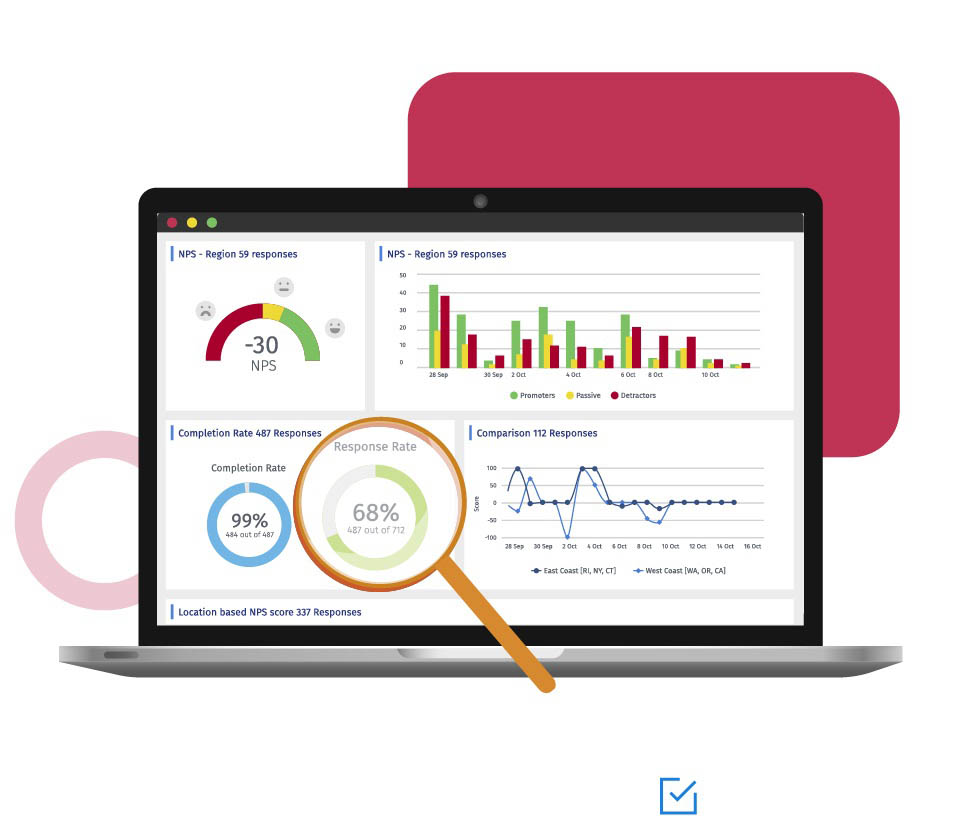# Correlation analysis

START A FREE TRIAL## What is correlation analysis?

Correlation analysis in research is a statistical method used to measure the strength of the linear relationship between two variables and compute their association. Simply put - correlation analysis calculates the level of change in one variable due to the change in the other.

A high correlation points to a strong relationship between the two variables, while a low correlation means that the variables are weakly related.

When it comes to market research, researchers use correlation analysis to analyze quantitative data collected through research methods like surveys and live polls. They try to identify the relationship, patterns, significant connections, and trends between two variables or datasets.

There is a positive correlation between two variabls when an increase in one variable leads to the increase in the other. On the other hand, a negative correlation means that when one variable increases, the other decreases and vice-versa.

## The Correlation Coefficient

One of the statistical concepts that is most related to this type of analysis is the correlation coefficient.

The correlation coefficient is the unit of measurement used to calculate the intensity in the linear relationship between the variables involved in a correlation analysis, this is easily identifiable since it is represented with the symbol r and is usually a value without units which is located between 1 and -1.

If you want to delve into this topic, we recommend you consult our guide: Pearson Correlation Coefficent.

## Example of correlation analysis

Correlation between two variables can be either a positive correlation, a negative correlation, or no correlation. Let's look at examples of each of these three types.

• Positive correlation: A positive correlation between two variables means both the variables move in the same direction. An increase in one variable leads to an increase in the other variable and vice versa.

For example, spending more time on a treadmill burns more calories.

• Negative correlation: A negative correlation between two variables means that the variables move in opposite directions. An increase in one variable leads to a decrease in the other variable and vice versa.

For example, increasing the speed of a vehicle decreases the time you take to reach your destination.

• Weak/Zero correlation: No correlation exists when one variable does not affect the other.

For example, there is no correlation between the number of years of school a person has attended and the letters in his/her name.## Uses of correlation analysis

Correlation analysis is used to study practical cases. Here, the researcher can't manipulate individual variables. For example, correlation analysis is used to measure the correlation between the patient's blood pressure and the medication used.

Marketers use it to measure the effectiveness of advertising. Researchers measure the increase/decrease in sales due to a specific marketing campaign.

In statistics, correlation refers to the fact that there is a link between various events. One of the tools to infer whether such a link exists is correlation analysis. Practical simplicity is undoubtedly one of its main advantages.

To perform reliable correlation analysis, it is essential to make in-depth observations of two variables, which gives us an advantage in obtaining results. Some of the most notorious benefits of correlation analysis are:

• Awareness of the behavior between two variables: A correlation helps to identify the absence or presence of a relationship between two variables. It tends to be more relevant to everyday life.

• A good starting point for research: It proves to be a good starting point when a researcher starts investigating relationships for the first time.

• Uses for further studies: Researchers can identify the direction and strength of the relationship between two variables and later narrow the findings down in later studies.

• Simple metrics: Research findings are simple to classify. The findings can range from -1.00 to 1.00. There can be only three potential broad outcomes of the analysis.

## How to use correlation analysis in your surveys?

Learn how to set up and use this feature with our help file on correlation analysis.

### Get started today!

#### Are you interested in using this feature? With QuestionPro you can gain access to this and many more. Do your data collection and research more efficiently than ever!

FREE 10-DAY TRIAL